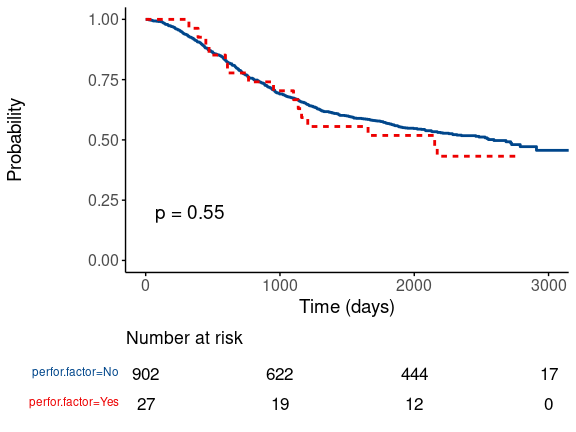# All plots examples

## 1 Odds ratio plots

### 1.01 Standard odds ratio plot (forest plot)

This is used to visually present the results from a multivariable generalised linear model (usually logistic regression).

Here is the model:

library(finalfit)
explanatory = c("age.factor", "sex.factor", "obstruct.factor", "perfor.factor")
dependent = 'mort_5yr'
colon_s %>%
finalfit(dependent, explanatory) %>%
knitr::kable(row.names=FALSE) # This line only needed for formatting. 
Dependent: Mortality 5 year Alive Died OR (univariable) OR (multivariable)
Age <40 years 31 (46.3) 36 (53.7) - -
40-59 years 208 (61.4) 131 (38.6) 0.54 (0.32-0.92, p=0.023) 0.57 (0.34-0.98, p=0.041)
60+ years 272 (53.4) 237 (46.6) 0.75 (0.45-1.25, p=0.270) 0.81 (0.48-1.36, p=0.426)
Sex Female 243 (55.6) 194 (44.4) - -
Male 268 (56.1) 210 (43.9) 0.98 (0.76-1.27, p=0.889) 0.98 (0.75-1.28, p=0.902)
Obstruction No 408 (56.7) 312 (43.3) - -
Yes 89 (51.1) 85 (48.9) 1.25 (0.90-1.74, p=0.189) 1.25 (0.90-1.76, p=0.186)
Perforation No 497 (56.0) 391 (44.0) - -
Yes 14 (51.9) 13 (48.1) 1.18 (0.54-2.55, p=0.672) 1.12 (0.51-2.44, p=0.770)

Simply switch to or_plot() for plot.

library(finalfit)
explanatory = c("age.factor", "sex.factor", "obstruct.factor", "perfor.factor")
dependent = 'mort_5yr'
colon_s %>%
or_plot(dependent, explanatory)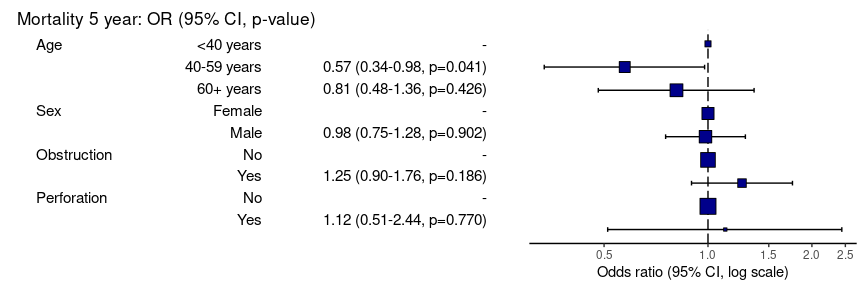# Note this example uses fig.height=3, fig.width=9

Adjust figure width and height to optimise formatting.

### 1.02 Mixed effects odds ratio plot (forest plot)

This is used to visually present the results from a mixed effects / multilevel / hierarchical generalised linear model (usually logistic regression).

Here is the model:

library(finalfit)
explanatory = c("age.factor", "sex.factor", "obstruct.factor", "perfor.factor")
dependent = 'mort_5yr'
random_effect = "hospital"
colon_s %>%
finalfit(dependent, explanatory, random_effect = random_effect)%>%
knitr::kable(row.names=FALSE) # This line only needed for formatting.
Dependent: Mortality 5 year Alive Died OR (univariable) OR (multilevel)
Age <40 years 31 (46.3) 36 (53.7) - -
40-59 years 208 (61.4) 131 (38.6) 0.54 (0.32-0.92, p=0.023) 0.75 (0.39-1.44, p=0.382)
60+ years 272 (53.4) 237 (46.6) 0.75 (0.45-1.25, p=0.270) 1.03 (0.55-1.96, p=0.916)
Sex Female 243 (55.6) 194 (44.4) - -
Male 268 (56.1) 210 (43.9) 0.98 (0.76-1.27, p=0.889) 0.80 (0.58-1.11, p=0.180)
Obstruction No 408 (56.7) 312 (43.3) - -
Yes 89 (51.1) 85 (48.9) 1.25 (0.90-1.74, p=0.189) 1.23 (0.82-1.83, p=0.320)
Perforation No 497 (56.0) 391 (44.0) - -
Yes 14 (51.9) 13 (48.1) 1.18 (0.54-2.55, p=0.672) 1.03 (0.43-2.51, p=0.940)
library(finalfit)
explanatory = c("age.factor", "sex.factor", "obstruct.factor", "perfor.factor")
dependent = 'mort_5yr'
random_effect = "hospital"
colon_s %>%
or_plot(dependent, explanatory, random_effect = random_effect)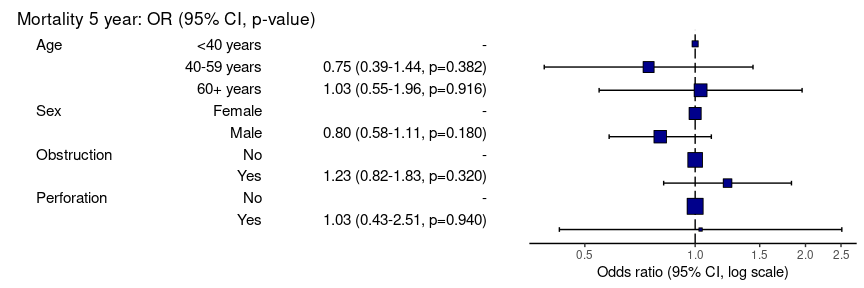# Note this example uses fig.height=3, fig.width=9

### 1.03 Plotting a subset of variables from a full model

You may wish to only present a subset of variables from a full model, say an explanatory variable of interest without covariates to avoid table 2 fallacy.

library(finalfit)
explanatory = c("age.factor", "sex.factor", "obstruct.factor", "perfor.factor")
dependent = 'mort_5yr'

# Run summary_factorlist for variables you wish to include
## Include total_col = TRUE and fit_id = TRUE
factorlist  = colon_s %>%
summary_factorlist(dependent, "age.factor", total_col = TRUE, fit_id = TRUE)

# Run full model including factorlist
colon_s %>%
or_plot(dependent, explanatory, factorlist = factorlist)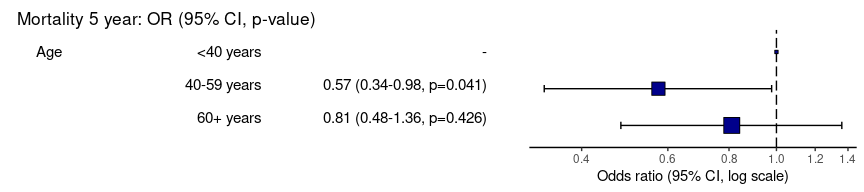# Note this example uses fig.height=2, fig.width=9

### 1.04 Plotting from model object

When a model takes a long time to run, you do not want to re-run it to plot it. Pass the model object to the plotting function.

library(finalfit)
explanatory = c("age.factor", "sex.factor", "obstruct.factor", "perfor.factor")
dependent = 'mort_5yr'
random_effect = "hospital"

fit = colon_s %>%
glmmixed(dependent, explanatory, random_effect)

# Equivalent to:
fit = colon_s %>%
lme4::glmer(mort_5yr ~ age.factor + sex.factor + obstruct.factor + perfor.factor + (1 | hospital),
family="binomial", data = .)

# Which is incidentally equivalent to:
fit = colon_s %>%
lme4::glmer(ff_formula(dependent, explanatory, random_effect),
family="binomial", data = .)

# Plot
system.time(colon_s %>%
or_plot(dependent, explanatory, random_effect = random_effect, glmfit = fit)
)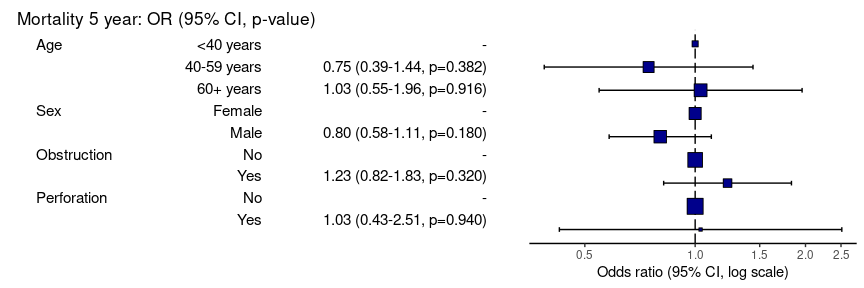#>    user  system elapsed
#>   0.528   0.004   0.537
# Note this example uses fig.height=3, fig.width=9

### 1.05 Confidence interval type

Change the type of confidence interval. For GLM, this is “profile” by default, but confusingly “default” is also available (Wald) which is quicker but may be biased when the likelihood function is not symmetrical around the maximum likelihood estimate. Translated - best to use “profile” or an alternative approach (like bootstrapping) for final results.

library(finalfit)
explanatory = c("age.factor", "sex.factor", "obstruct.factor", "perfor.factor")
dependent = 'mort_5yr'
colon_s %>%
or_plot(dependent, explanatory, confint_type = "default")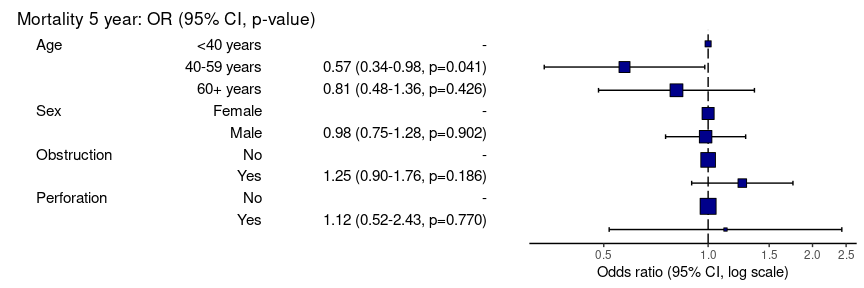# Note this example uses fig.height=3, fig.width=9

### 1.06 Remove reference levels

This will remove reference levels for binary variables.

library(finalfit)
explanatory = c("age.factor", "sex.factor", "obstruct.factor", "perfor.factor")
dependent = 'mort_5yr'
colon_s %>%
or_plot(dependent, explanatory, remove_ref = TRUE)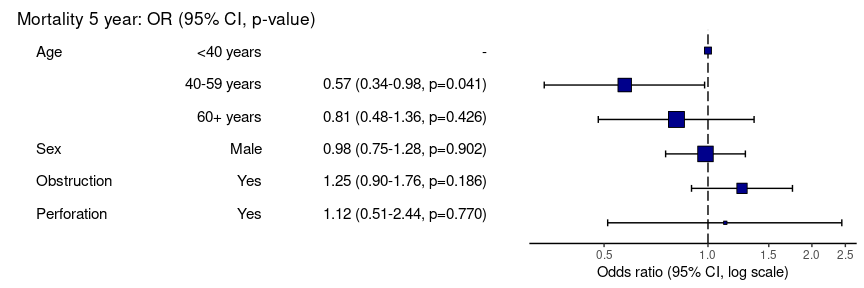# Note this example uses fig.height=3, fig.width=9

### 1.07 Manually specifiy x-axis breaks

Note, this will not include labels that are outwith the axis range, i.e., if the axis starts at 0.2, adding 0.1 will not result in any change. Use plot_opts to alter axis range.

library(finalfit)
explanatory = c("age.factor", "sex.factor", "obstruct.factor", "perfor.factor")
dependent = 'mort_5yr'
colon_s %>%
or_plot(dependent, explanatory, breaks = c(0.4, 0.6, 0.8, 1.0, 1.2, 1.4, 1.8, 2.4))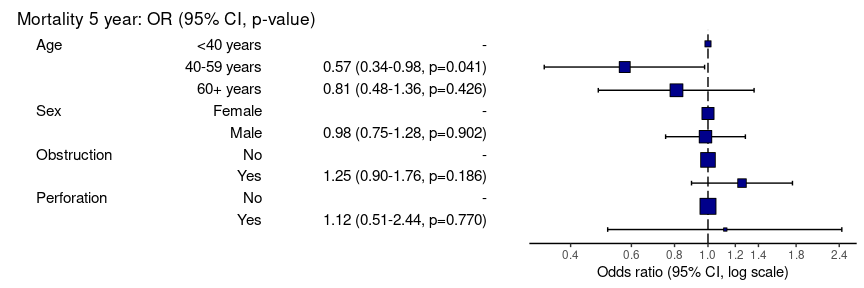# Note this example uses fig.height=3, fig.width=9

### 1.08 Adjust table column spacing

Nudge relative column positions to manage white space. Use in combination with figure width to optimise.

library(finalfit)
explanatory = c("age.factor", "sex.factor", "obstruct.factor", "perfor.factor")
dependent = 'mort_5yr'
colon_s %>%
or_plot(dependent, explanatory, column_space = c(-0.5, -0.1, 0.5))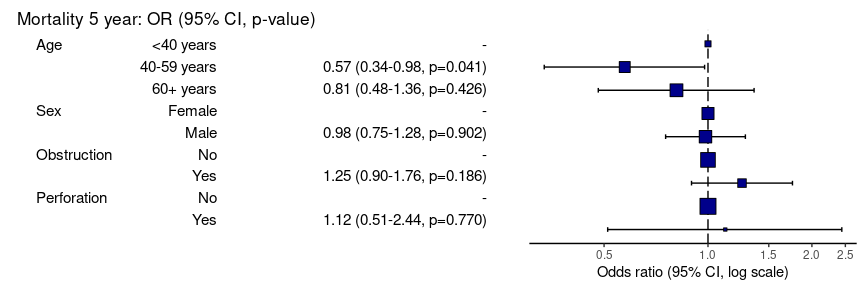# Note this example uses fig.height=3, fig.width=9

### 1.09 Adjust or remove dependent variable label

library(finalfit)
explanatory = c("age.factor", "sex.factor", "obstruct.factor", "perfor.factor")
dependent = 'mort_5yr'
colon_s %>%
or_plot(dependent, explanatory, dependent_label = "Mortality")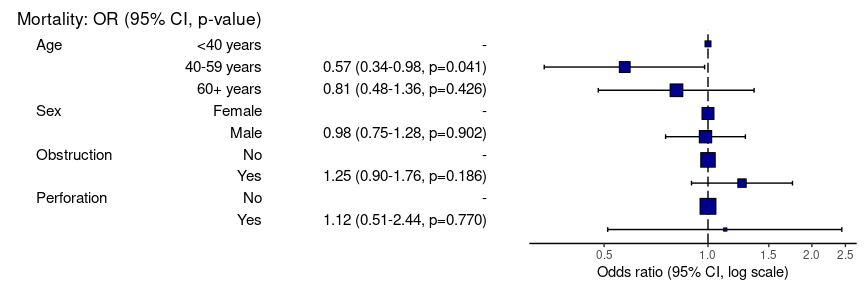# Note this example uses fig.height=3, fig.width=9

### 1.10 Add dependent variable label prefix

library(finalfit)
explanatory = c("age.factor", "sex.factor", "obstruct.factor", "perfor.factor")
dependent = 'mort_5yr'
colon_s %>%
or_plot(dependent, explanatory, prefix = "Figure 1 - ")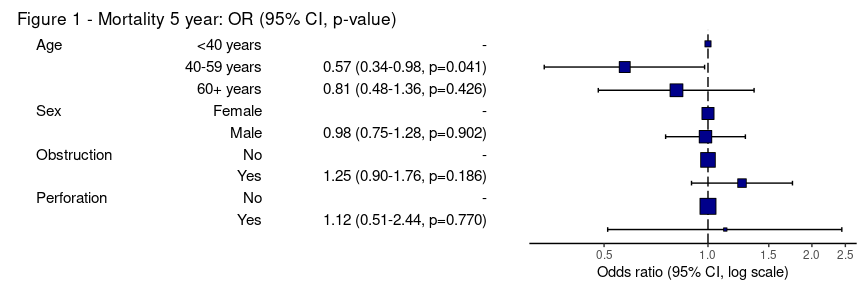# Note this example uses fig.height=3, fig.width=9

### 1.11 Adjust or remove dependent variable label suffix

library(finalfit)
explanatory = c("age.factor", "sex.factor", "obstruct.factor", "perfor.factor")
dependent = 'mort_5yr'
colon_s %>%
or_plot(dependent, explanatory, suffix = "")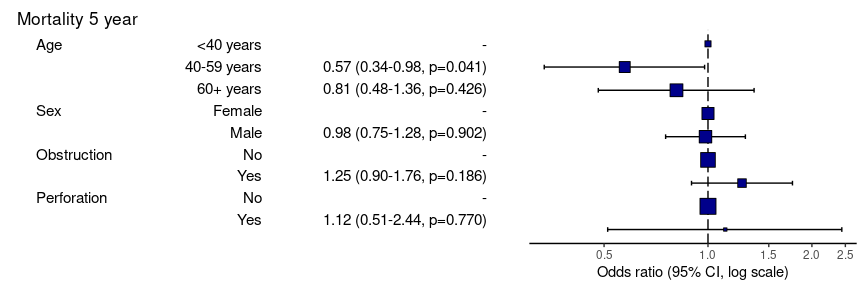# Note this example uses fig.height=3, fig.width=9

### 1.12 Adjust table text size

library(finalfit)
explanatory = c("age.factor", "sex.factor", "obstruct.factor", "perfor.factor")
dependent = 'mort_5yr'
colon_s %>%
or_plot(dependent, explanatory, table_text_size = 3)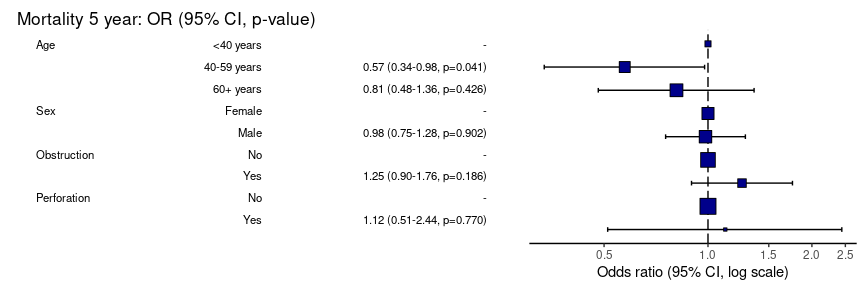# Note this example uses fig.height=4, fig.width=9

### 1.13 Adjust title text size

library(finalfit)
explanatory = c("age.factor", "sex.factor", "obstruct.factor", "perfor.factor")
dependent = 'mort_5yr'
colon_s %>%
or_plot(dependent, explanatory, title_text_size = 12)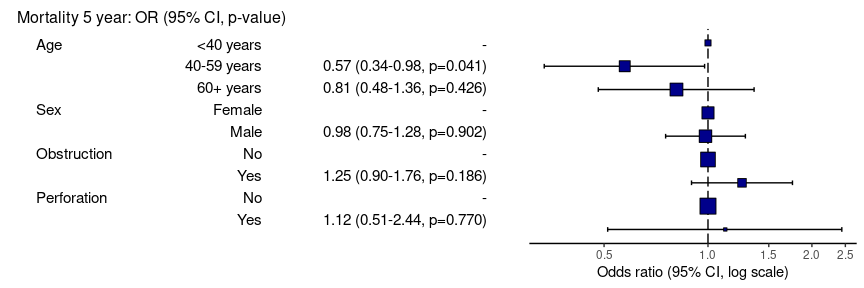# Note this example uses fig.height=3, fig.width=9

This adds further ggplot arguments appended in the usual way by “+”.

library(finalfit)
library(ggplot2)
explanatory = c("age.factor", "sex.factor", "obstruct.factor", "perfor.factor")
dependent = 'mort_5yr'
colon_s %>%
or_plot(dependent, explanatory,
plot_opts = list(xlim(0.1, 3),
xlab("OR (95% CI, log)"),
theme(axis.title = element_text(size=10))
)
)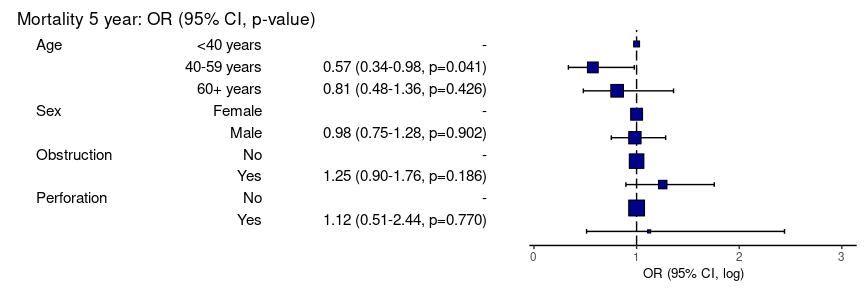# Note this example uses fig.height=3, fig.width=9

Pass any options for fit2df() to adjust table, e.g., number of decimal places, confidence interval separator etc.

library(finalfit)
explanatory = c("age.factor", "sex.factor", "obstruct.factor", "perfor.factor")
dependent = 'mort_5yr'
colon_s %>%
or_plot(dependent, explanatory,
digits = c(3,3,3), confint_sep = " to ")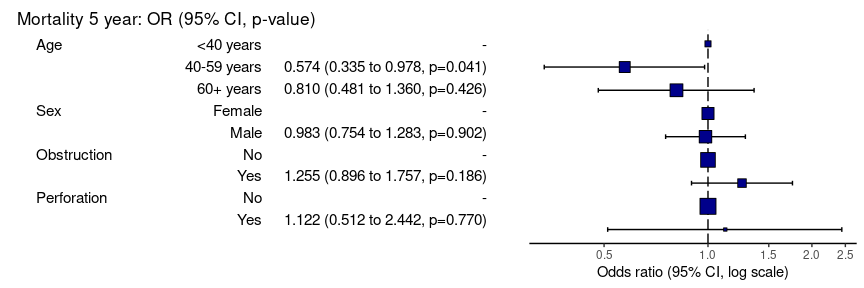# Note this example uses fig.height=3, fig.width=9

## 2 Hazard ratio plots

### 2.01 Standard hazard ratio plot

This is used to visually present the results from a multivariable Cox Proportional Hazards model.

Here is the model:

library(finalfit)
explanatory = c("age.factor", "sex.factor", "obstruct.factor", "perfor.factor")
dependent = "Surv(time, status)"
colon_s %>%
finalfit(dependent, explanatory) %>%
knitr::kable(row.names=FALSE) # This line only needed for formatting. 
Dependent: Surv(time, status) all HR (univariable) HR (multivariable)
Age <40 years 70 (7.5) - -
40-59 years 344 (37.0) 0.76 (0.53-1.09, p=0.132) 0.79 (0.55-1.13, p=0.196)
60+ years 515 (55.4) 0.93 (0.66-1.31, p=0.668) 0.98 (0.69-1.40, p=0.926)
Sex Female 445 (47.9) - -
Male 484 (52.1) 1.01 (0.84-1.22, p=0.888) 1.02 (0.85-1.23, p=0.812)
Obstruction No 732 (80.6) - -
Yes 176 (19.4) 1.29 (1.03-1.62, p=0.028) 1.30 (1.03-1.64, p=0.026)
Perforation No 902 (97.1) - -
Yes 27 (2.9) 1.17 (0.70-1.95, p=0.556) 1.08 (0.64-1.81, p=0.785)

Simply switch to hr_plot() for plot.

library(finalfit)
explanatory = c("age.factor", "sex.factor", "obstruct.factor", "perfor.factor")
dependent = "Surv(time, status)"
colon_s %>%
hr_plot(dependent, explanatory)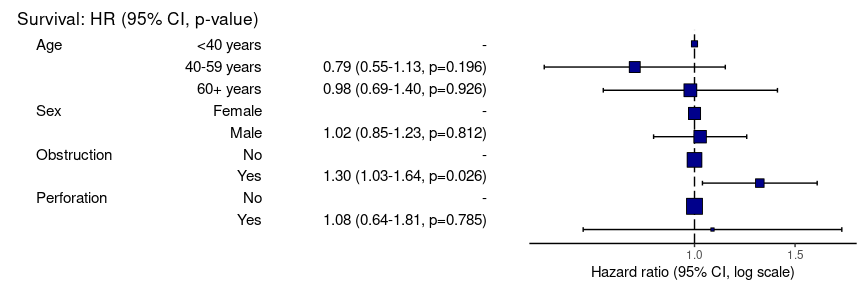# Note this example uses fig.height=3, fig.width=9
• All options list for odds ratio plots above (section 1) apply to hazard ratio plots.

## 3 Coefficient plots

### 3.01 Standard coefficient plots

This is used to visually present the results from a multivariable linear regression model.

#’ # Coefficient plot #’ explanatory = c(“age.factor”, “sex.factor”, “obstruct.factor”, “perfor.factor”) #’ dependent = “nodes” #’ colon_s %>% #’ coefficient_plot(dependent, explanatory)

Here is the model:

library(finalfit)
explanatory = c("age.factor", "sex.factor", "obstruct.factor", "perfor.factor")
dependent = "nodes"
colon_s %>%
finalfit(dependent, explanatory) %>%
knitr::kable(row.names=FALSE) # This line only needed for formatting. 
Dependent: nodes unit value Coefficient (univariable) Coefficient (multivariable)
Age <40 years Mean (sd) 4.7 (4.5) - -
40-59 years Mean (sd) 3.6 (3.3) -1.14 (-2.08 to -0.21, p=0.016) -1.21 (-2.16 to -0.26, p=0.012)
60+ years Mean (sd) 3.6 (3.6) -1.19 (-2.10 to -0.28, p=0.010) -1.25 (-2.18 to -0.33, p=0.008)
Sex Female Mean (sd) 3.7 (3.6) - -
Male Mean (sd) 3.6 (3.6) -0.14 (-0.60 to 0.33, p=0.565) -0.07 (-0.54 to 0.40, p=0.779)
Obstruction No Mean (sd) 3.7 (3.7) - -
Yes Mean (sd) 3.5 (3.2) -0.24 (-0.83 to 0.36, p=0.435) -0.31 (-0.91 to 0.29, p=0.313)
Perforation No Mean (sd) 3.7 (3.6) - -
Yes Mean (sd) 3.9 (2.8) 0.24 (-1.13 to 1.61, p=0.735) 0.28 (-1.09 to 1.66, p=0.686)

Simply switch to coeffcient_plot() for plot.

library(finalfit)
explanatory = c("age.factor", "sex.factor", "obstruct.factor", "perfor.factor")
dependent = "nodes"
colon_s %>%
coefficient_plot(dependent, explanatory)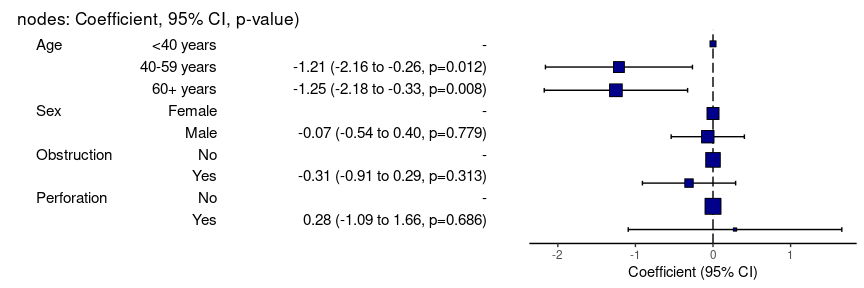# Note this example uses fig.height=3, fig.width=9
• All options list for odds ratio plots above (section 1) apply to coefficient ratio plots.

## 4 Wrapper for all plot types

ff_plot() will automatically determine the dependent variable type (binary, survival object, or continuous), and produce the appropriate output (odds ratio, hazard ratio, or coefficient plot).

library(finalfit)
explanatory = c("age.factor", "sex.factor", "obstruct.factor", "perfor.factor")
dependent = "nodes"
colon_s %>%
ff_plot(dependent, explanatory)# Note this example uses fig.height=3, fig.width=9

## 5 Kaplan Meier plots

Use finalfit grammar to plot a survival curve with number-at-risk table using survminer::ggsurvplot().

### 5.1 Standard KM plot

library(finalfit)
explanatory = "perfor.factor"
dependent = "Surv(time, status)"
colon_s %>%
surv_plot(dependent, explanatory)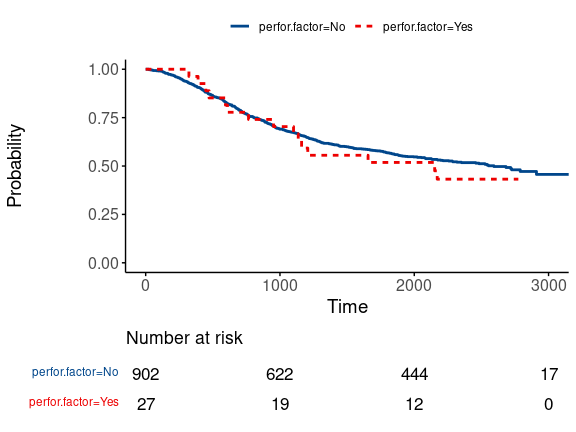Pass any of the options from survminer::ggsurvplot().

For example:

library(finalfit)
explanatory = "perfor.factor"
dependent = "Surv(time, status)"
colon_s %>%
surv_plot(dependent, explanatory, xlab="Time (days)", pval=TRUE, legend="none")